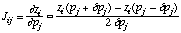# Manual Page for Command >>> CENTERED

Syntax
>>> CENTERED

Parent Command
>> JACOBIAN

Subcommand
-

Description
This command calculates elements of the Jacobian matrix by means of centered finite difference quotients:The evaluation of the Jacobian thus requires (2n+1) TOUGH2 simulations, where n is the number of parameters. The size of the perturbation dp can be controlled using command >&gt;> PERTURB. Centered finite differences are more accurate than forward finite differences, but computationally twice as expensive (also see command >>> FORWARD).

Example
> COMPUTATION
>> JACOBIAN
>>> use CENTERED finite difference quotient
>>> PERTURBation factor is : 0.005 times the parameter value
<<<
<<